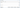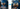•• Merch
• Donate
• SAT Prep

Function Composition CalculatorFunction Composition Calculator# Function Composition

### Function Inputs & Outputs

When you see something like or , you have function composition.

First off, think of handling functions like doing a find & replace.When we have a function like plugging an input in for means finding & replacing every with the input value. For example, if our input is , we find & replace to get:
⬇️
And you have to make sure you replace every It's like how they had to replace every creepy Sonic with the new, cute Sonic, otherwise the creepiness would still be there ☠️.If you need more help with functions, check out our functions lesson and calculator here!

### What is Function Composition?

The idea behind function composition is that instead of a number, another function acts as the input.

So, let's say we have these two functions:

If we're asked for then our input is and we need to replace every in with
⬇️
And since we know that , we can simplify by using instead of on the right side:
And that's how we get

### Plugging in a Value

Now, if we're asked for instead of then we need to do another find & replace to replace every in our new equation with our input value of
⬇️
Then we have all numbers on the right side of the equation, and we can just calculate to find our final answer of

### Graphing Function Composition

You might get the functions in graph forms, like this:
In this case, we use our graphs to find the function composition. Let's say we're asked for
This means, first we need to find what is. Let's look at our graph for where our
Looking at the point on the line where we can see that the value is This means that
Now, if we go back to our original composition of we can replace with to get
Now, we just look at our graph of at the point where
Looking at the point on the line where we can see that the value is This means that
So to review, to solve for we first needed to find the value of Once we found that we replaced with to get:
Looking at the graph for we find:

#### Function Composition through Graphs

Let's say we're looking for

#### Step 1: Find the inner value.

First, we use the graph for to find the value of

#### Step 2: Plug in the inner value.

Next, we plug in the value we found into the composition. Let's say we found that our value was We plug that into our composition to replace to get

#### Step 3: Find the final value.

Finally, we use the graph for to find the value of Once we do that, we're done!